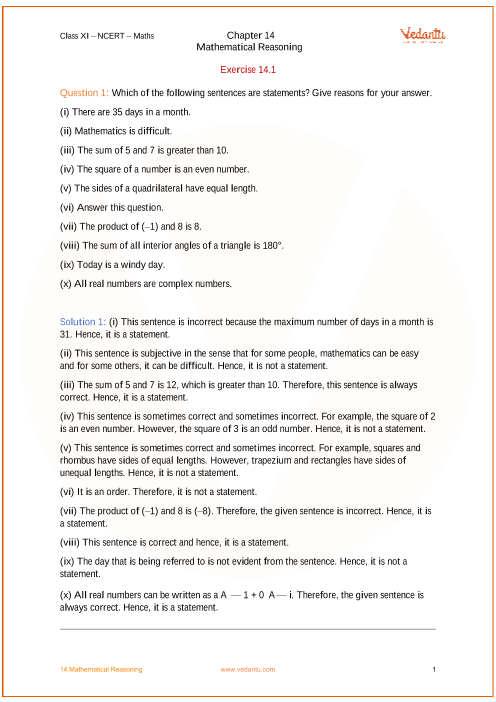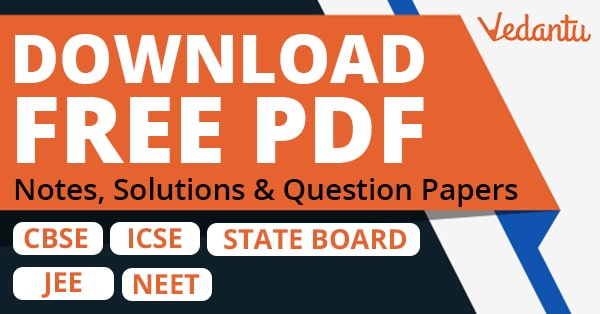# NCERT Solutions for Class 11 Maths Chapter 14 Mathematical Reasoning (Ex 14.2) Exercise 14.2

## NCERT Solutions for Class 11 Maths Chapter 14 Mathematical Reasoning (Ex 14.2) Exercise 14.2

Free PDF download of NCERT Solutions for Class 11 Maths Chapter 14 Exercise 14.2 (Ex 14.2) and all chapter exercises at one place prepared by expert teacher as per NCERT (CBSE) books guidelines. Class 11 Maths Chapter 14 Mathematical Reasoning Exercise 14.2 Questions with Solutions to help you to revise complete Syllabus and Score More marks. Register and get all exercise solutions in your emails.